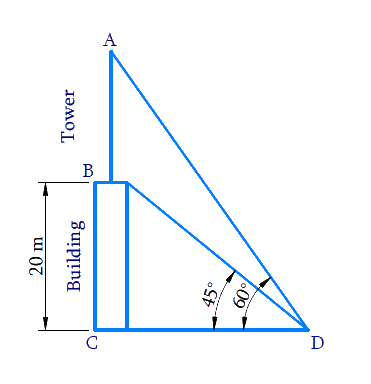# Ex.9.1 Q7 Some Applications of Trigonometry Solution - NCERT Maths Class 10

Go back to  'Ex.9.1'

## Question

From a point on the ground, the angles of elevation of the bottom and the top of a transmission tower fixed at the top of a $$20\,\rm{m}$$ high building are $$45^\circ$$ and $$60^\circ$$ respectively. Find the height of the tower.

Video Solution
Some Applications Of Trigonometry
Ex 9.1 | Question 7

## Text Solution

What is Known?

(i) Angle of elevation from ground to bottom of the tower $$= 45^\circ$$

(ii) Angle of elevation from ground to top of the tower $$= 60^\circ$$

(iii) Height of the building $$= 20 \,\rm{m}$$

(iv) Tower is fixed at the top of the $$20\,\rm{m}$$ high building

What is Unknown?

Height of the towerReasoning:

Let the height of the building is $$BC$$, height of the transmission tower which is fixed at the top of the building is $$AB$$. $$D$$ is the point on the ground from where the angles of elevation of the bottom $$B$$ and the top $$A$$ of the transmission tower $$AB$$ are $$45^\circ$$ and $$60^\circ$$ respectively.

The distance of the point of observation $$D$$ from the base of the building $$C$$ is $$CD$$

Combined height of the building and tower $$= AC = AB + BC$$

Trigonometric ratio involving sides $$AC,\, BC, \,CD$$ and $$∠D\, (45^\circ \rm{and}\, 60^\circ)$$ is $$\tan \theta$$

Steps:

In $$\Delta {BCD}$$

\begin{align}\tan 45^{\circ} &=\frac{B C}{C D} \\ 1 &=\frac{20}{C D} \\ C D &=20 \mathrm{m} \end{align}

In  $$\Delta {ACD}$$

\begin{align} \tan 60^{\circ} &=\frac{A C}{C D} \\ \sqrt{3} &=\frac{A C}{20} \\ A C &=20 \sqrt{3} \mathrm{m} \end{align}

Height of the tower,

$$AB = AC - BC$$

\begin{align}AB&=20\sqrt{3}\text m-20\text m \\ & =20\left( \sqrt{3}-1 \right)\rm m \end{align}

Learn from the best math teachers and top your exams

• Live one on one classroom and doubt clearing
• Practice worksheets in and after class for conceptual clarity
• Personalized curriculum to keep up with school